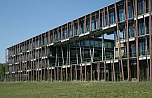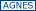# Humboldt Universitaet zu BerlinFaculty of Mathematics and Natural SciencesDepartment of Physicsauf deutsch

alles unter Vorbahlt von COVID-19 Maßnahmen
Beachten Sie die Hinweise in den jeweligen Veranstaltungen

summer sem. 2021
Last update: 13.12.21 21:39:34

_SS 2022 WS 2021
SS 2021
SS 2020 WS 2020
Department of Physics
open chemistry

### 4020210077 Topological recursion, matrix models and intersection theoryVL Mon 9-11 weekly nV or digital (0) Gaétan Borot Wed 9-11 weekly nV or digital (0) UE Mon 11-13 weekly nV or digital (0) Gaétan Borot

Digital- & Präsenz-basierter Kurs

classroom language
DE
aims
The topological recursion is a universal recursion, whose structure resembles the recursive construction of surfaces of any topology by cutting and pasting, and governs diverse problems in mathematics and physics. The first part of the course will introduce the recursion, discuss its algebraic meaning from two perspectives (quantization in affine symplectic spaces, geometry of Riemann surfaces) and study its essential properties. The second part will show how it can be applied to asymptotic expansion in random matrix theory, to the enumeration of branched covers of the sphere (Hurwitz theory) and to intersection theory on the moduli space of complex curves.
requirements
Prerequisites are a good command of complex analysis (as well as differential geometry for Part V). Prior knowledge in random matrix theory and on the moduli space of curves is not necessary.
structure / topics / contents
I. Airy structures, partition function, quantization of quadratic Lagrangian in affine symplectic spaces and its relation to the topology of surfaces, properties. Basic examples and constructions from the Virasoro algebra.

II. Spectral curves and topological recursion. Abstract loop equations and their solution by the topological recursion. Form cycle duality, variation of initial data and special geometry, holomorphic anomaly equation. Prepotential.

III. Formal and convergent matrix integrals. Schwinger-Dyson equations. The large size limit and the spectral curve. Existence of all-order asymptotic expansion in the size. Relation to loop equations and solution by the topological recursion.

IV. Hurwitz theory: branched covers of surfaces, representation of the symmetric group, enumeration problem. Cut and join equations, relation to loop equations, solution by the topological recursion. Glimpse into mirror symmetry.

V. The moduli space of curves, tautological classes. psi-classes. Combinatorial model of the moduli space and its geometry. Mirzakhani-McShane type-identities and topological recursion. Witten-Kontsevich theorem. General representation of the output of topological recursion via intersection theory. Application: ELSV formula for Hurwitz numbers. Cohomological field theories, TQFTs, Givental group action. Teleman's classification.
assigned modules
P25.1.b
amount, credit points; Exam / major course assessment
6 SWS, 6 SP/ECTS (Arbeitsanteil im Modul für diese Lehrveranstaltung, nicht verbindlich)
Anfragen/Probleme executed on vlvz1 © IRZ Physik, Version 2019.1.1 vom 24.09.2019 Fullscreen